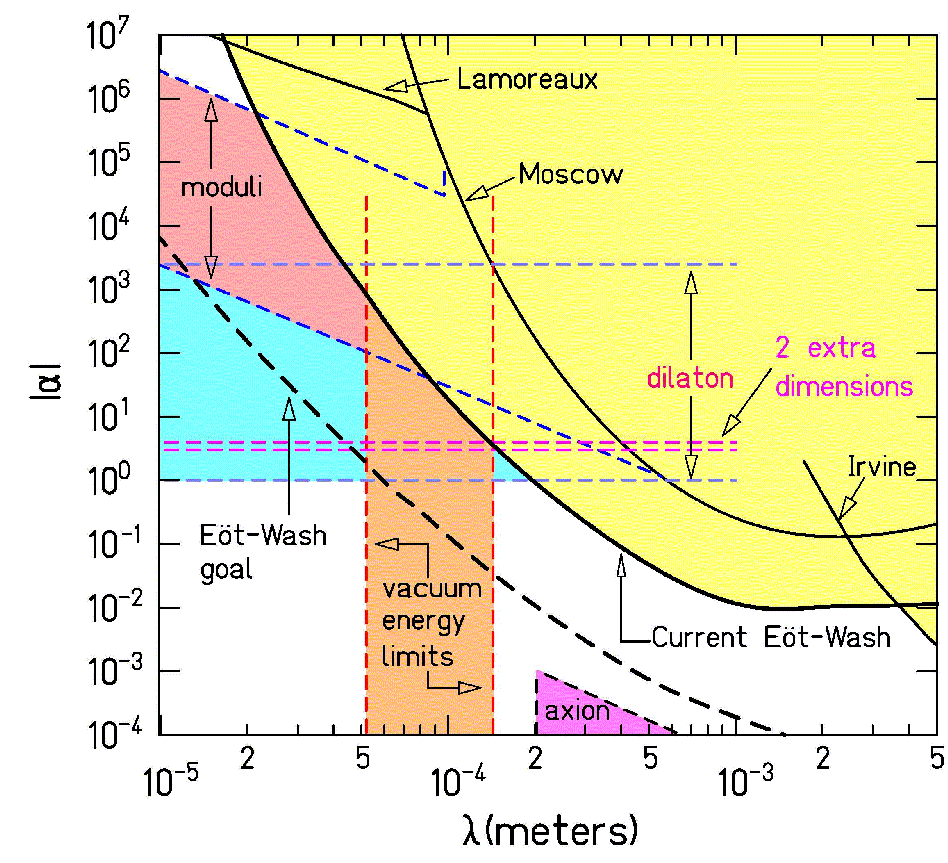# Results

 The plot on the right shows 95% confidence limits on equivalence-principle-violating Yukawa interactions as a function of Yukawa range. This plot assumes that the interaction couples to baryon number, a conservative case where all test bodies have very similar "charge"/mass ratios.The horizontal axes show the Yukawa range or alternatively the exchanged boson mass; the vertical axes show the Yukawa interaction's "fine structure constant" Ã— 10 40 or alternatively the dimensionless parameter defined so that the Yukawa force has a strength relative to gravity of alpha Ã— where (which has a numerical value of about 2 Ã— 10-3) measures the difference in "charge"/mass ratios of the test bodies. For long ranges, the force sensitivity is about 1 Ã— 10-12 of gravity. We now have obtained differential acceleration sensitivities of about 4 Ã— 10-13 cm/s2. One can appreciate how small this is by noting that if an object, initially at rest, had maintained that acceleration since the time of Pericles, it would now be moving about as fast as the end of the minute hand on a standard wall clock.

 This plot shows the 95% confidence level constraints on a Yukawa violation of the gravitational inverse-square law (as of 1/17/02). The vertical axis represents the strength of a deviation relative to that of Newtonian gravity while the horizontal axis designates its characteristic range. The yellow region has been excluded. The region excluded by our experiments has been updated since the publication of our paper . These results include an improved analysis of the published data, as well as data from an additional experiment.(click for enlarged image)

The results constrain extra dimensions to be smaller than 150 um, for the particular case that there are two large extra dimensions of equal size (see the paper by Arkani-Hamed, Dimopoulos, and Dvali for more information). A detailed description of this analysis and its implications is given in our paper. The dashed curve represents the area of parameter space we will be able to explore with our next-generation apparatus within the near future. References for the various theoretical and experimental curves can be found in our paper .